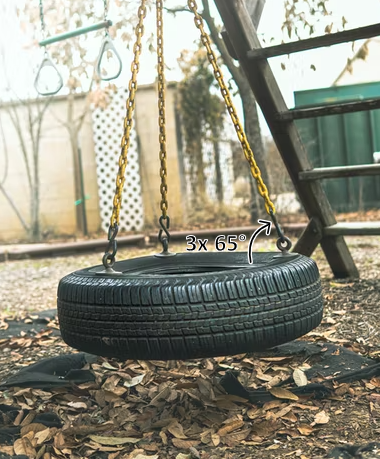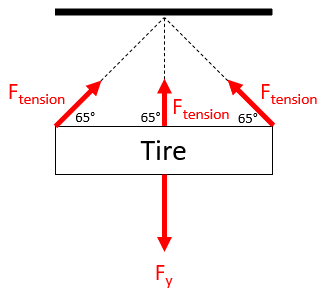## Tire Swing

Consider a tire swing found in a typical playground is suspended via 3 chain-link cables that experience a 90 N tensional force when a small child sits on the tire. If the angle formed between each cable and the tire is 65°, what is the child’s mass in kg? Ignore the weight of the tire and chain-link cables.Hint
The problem is asking to solve for $$F_{y}$$ in the free body diagram:Hint 2
Newton’s 2nd law:
$$F_y=mass\times acceleration$$$The problem is asking to solve for $$F_{y}$$ in the free body diagram:Because there are three upward pulling cables in the vertical direction, they will counteract the entire downward force. Keep in mind that $$F_{tension}$$ has both a x-component and y-component, but only the vertical component is relevant to this situation. Thus: $$F_y=(3)F_{tension}\cdot sin(65^{\circ})=(3)(90N)\cdot sin(65^{\circ})=244.7\:N$$$
To find the child’s mass, recall Newton’s 2nd law:
$$F_y=mass\times acceleration$$$$$mass=\frac{F_y}{gravity}=\frac{244.7N}{9.8\frac{m}{s^2}}=\frac{244.7kg\cdot \frac{m}{s^2}}{9.8\frac{m}{s^2}}=25\:kg$$$
25 kg